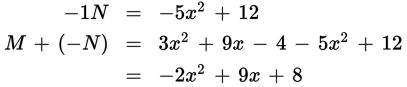# SAT Math Multiple Choice Question 410: Answer and Explanation

### Test Information

Question: 410

5. If M = 3x2 + 9x - 4 and N = 5x2 - 12, then what is 2(M - N)?

• A. -2x2 + 9x + 8
• B. -4x2 + 18x - 32
• C. -4x2 + 18x + 16
• D. 8x2 + 9x - 16

Explanation:

C

Difficulty: Medium

Category: Passport to Advanced Math / Exponents

Strategic Advice: Adding polynomials is typically safer than subtracting them, because you may forget to distribute the negative sign when subtracting more than one term.

Getting to the Answer: To find M - N, multiply each term of N by -1 and then add the two polynomials by combining like terms.Don't forget to multiply the resulting expression by 2 to get 2(-2x2 + 9x + 8) = -4x2 + 18x + 16.